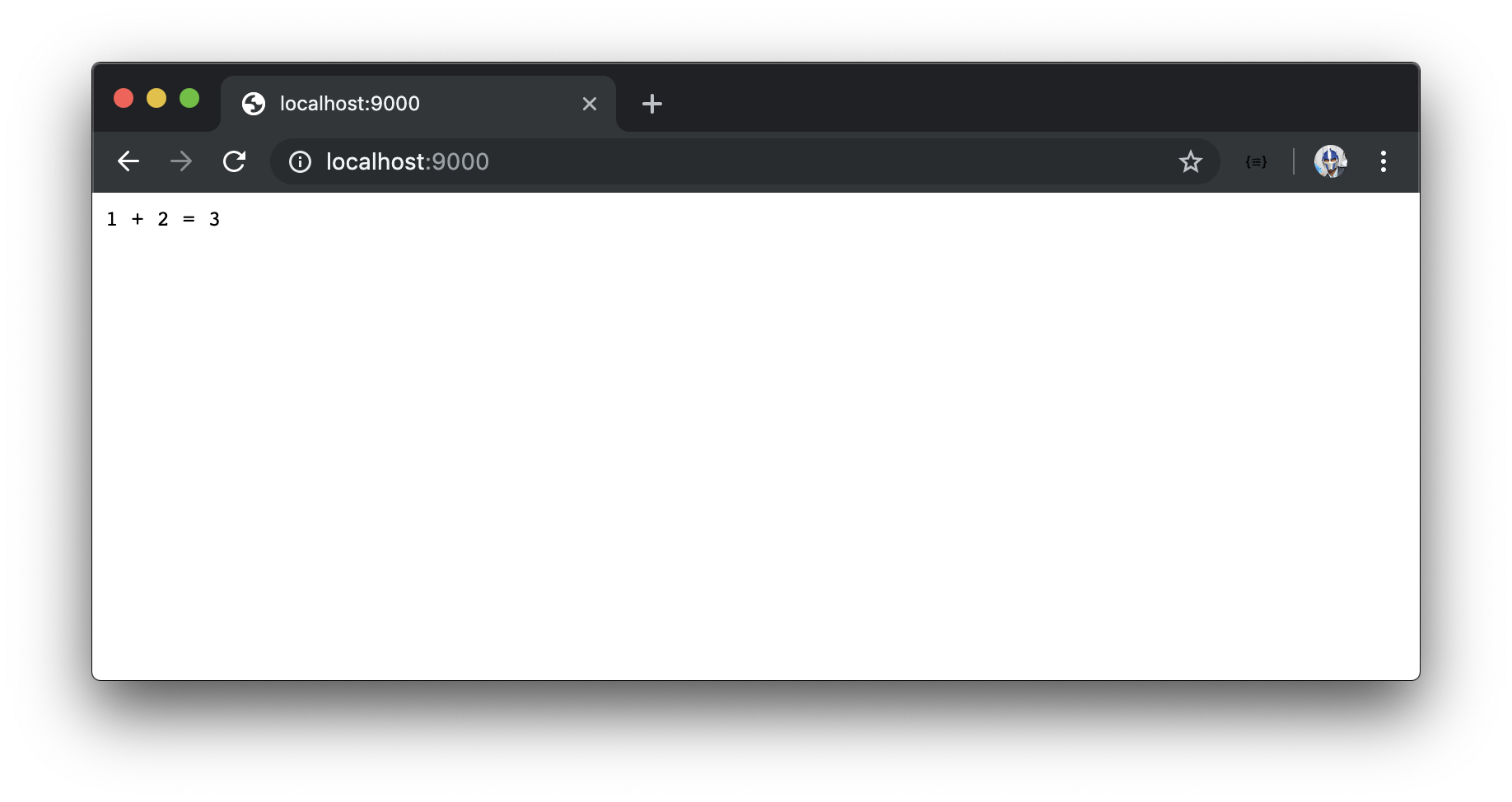This video is available to students only

# Introducing TypeScript

JavaScript is considered a weakly typed language. In this lesson, we'll go through a simple example of why that can be an issue in development and where TypeScript falls in the picture.

# Introducing TypeScript#

📝 This lesson's quiz can be found - here.
🗒️ Solutions for this lesson's quiz can be found - here.

JavaScript is considered a weakly typed language which means that in JavaScript, we have the ability to assign one data type to a variable and later on assign another data type to that same variable.

Let's see an example of this in our `index.js` file. We'll look to replicate what we've done before by creating two variables, `one` and `two`, and attempt to show the summation of these two variables.

In the `src/index.js` of our server project, we'll create constant variables labeled `one` and `two` and provide numerical values of `1` and `2` respectively.

``````const one = 1;
const two = 2;
``````

In the callback function of our `app.get()` method for the index route, we'll send an interpolated string that says `1` plus `2` is equal to the sum of the variables `one` and `two`.

server/src/index.js
``````app.get("/", (req, res) => res.send(`1 + 2 = \${one + two}`));
``````

When we launch our app in http://localhost:9000/, we'll see an output of 1 + 2 = 3.What if shortly after we've instantiated our constant variables, we decide to reassign the `two` variable to be a string with a value of 'two'. Since we're reassigning the value of a variable - we'll change how we instantiate the variable by using the `let` keyword.

Please select a discussion on the left.